## A positive charge +q1 is located to the left of a negative charge -q2. On a line passing through the two charges, there are two places where

Question

A positive charge +q1 is located to the left of a negative charge -q2. On a line passing through the two charges, there are two places where the total potential is zero. The first place is between the charges and is 1.0 cm to the left of the negative charge. the second place is 5.2 cm to the right of the negative charge.

(a) What is the distance between the charges?
___________cm
(b) Find q1/q2, the ratio of the magnitudes of the charges.
q1/q2 = ____________

in progress 0
2 months 2021-07-24T01:26:38+00:00 2 Answers 15 views 0

Explanation:

(a) If we assume that our origin of coordinates is at the position of charge q1, we have that the potential in both points is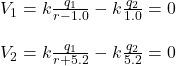k=8.89*10^9

For both cases we have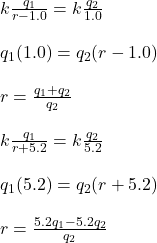(b) by replacing this values of r in the expression for V we obtain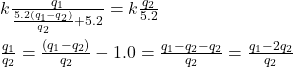hope this helps!!

a) d = 2.48 cm

b)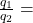1.48 cm

Explanation:

The potential at the point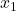= 1.0 cm to the left of the charge is given as: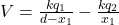since V = 0 ; Then: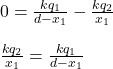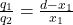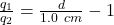The potential at the point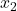= 5.2  cm to the right  of the negative charge is: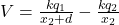since V = 0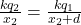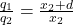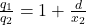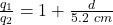Now, let’s solve for d (the distance between the charges ) from the above derived formulas

If we represent the ratio of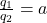Then;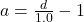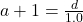1.0a + 1 = d      ——- equation (1)

Also;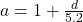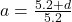5.2 a = 5.2 +d

5.2 a – 5.2 = d      ———- equation (2)

From equation (1) ; lets replace  d = 5.2 a – 5.2

then :

1.0 a + 1 = d

1.0 a + 1 = 5.2 a – 5.2

1.0a – 5.2 a = – 5.2 – 1

– 4.2 a = -6.2

a = 1.48 cm

Also replace a = 1.48 cm into equation (1) to solve for d

1.0 a + 1 = d

1.0 (1.48 )+ 1  = d

1.48 + 1 = d

d = 2.48 cm

b)

The ratio of the magnitude of the charges :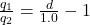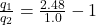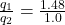1.48 cm